# Magic Star Examples-2

This page contains examples of magic stars from order-12 to order-14. Because there are multiple patterns for each order  there are a total of 14 different magic star examples.

The Magic Star Examples page shows the 14 patterns for orders 5 to 11.

### Contents

 Introduction. . to magic stars Order-12a ....# 275 of > 800,000 Order-12b ....# 397829 of > 800,000 Order-12c ....# 325000 of > 800,000 Order-12d ....# 277030 of > 800,000 Order-13a ....# 10 of > 3,000,000 Order-13b ....# 10 of > 3,000,000 Order-13c ....# 10 of > 3,000,000 Order-13d ....# 10 of > 3,000,000 Order-13e ....# 10 of > 3,000,000 Order-14a ....# 10 of > 7,000,000? Order-14b ....# 10 of > 7,000,000? Order-14c ....# 10 of > 7,000,000? Order-14d ....# 10 of > 7,000,000? Order-14e ....# 10 of > 7,000,000?

## Example Magic Stars

###Order-12 uses the consecutive numbers from 1 to 24
(2 x 12).

Patterns A, B and C are non-continuous, pattern D is continuous.

Index # 475? of estimated 800,000+ basic solutions. Each line sums to 50.

This pattern contains two super-imposed hexagons (12 = 2 x 6). In this case the corners of each hexagon sum to 60. In all cases the corners of the two hexagons will sum to the same value.###Index # 397829 of 826112 basic solutions.
It's points contain the numbers from 1 to 12.
Its complement is # 826112,  the last basic solution in the list, and obviously has the numbers 13 to 24 at the points.

My 200 Mhz Pentium Pro, with 64 Megs of RAM took 39.5 days to find the 826112 basic solutions. The data file size is  50 Megabytes.

This pattern contains three super-imposed squares (12 = 3 x 4).

###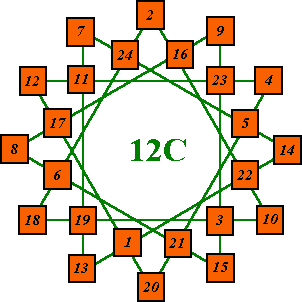Index # 325,000 of estimated 800,000+ basic solutions. Each line sums to 50.

This pattern contains four super-imposed triangles
(12 = 4 x 3). Their points sum to 30, 32, 34, 36.###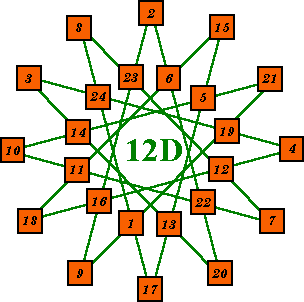Index # 277030 of estimated 800,000+ basic solutions. Each line sums to 50.

This pattern is the only one of four for this order that is continuous.

I

### Order-13A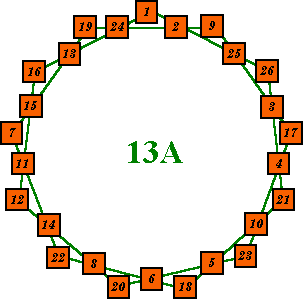Order-13 uses the numbers from 1 to 26. Each of the 13 lines sums to 54.

There are five different patterns in this order and because 13 is a prime number, all 5 patterns are continuous.

This is index #10 of more then 3,000,000 basic solutions for order-13A.### Order-13B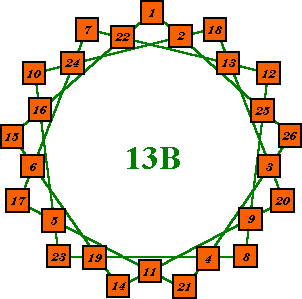This is index #10 of more then 3,000,000 basic solutions for order-13B.

### Order-13C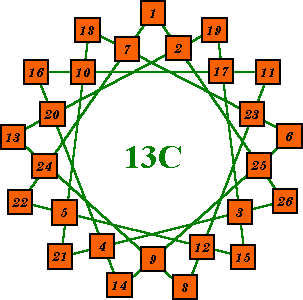This is index #10 of more then 3,000,000 basic solutions for order-13C.### Order-13DThis is index #10 of more then 3,000,000 basic solutions for order-13D.

### Order-13E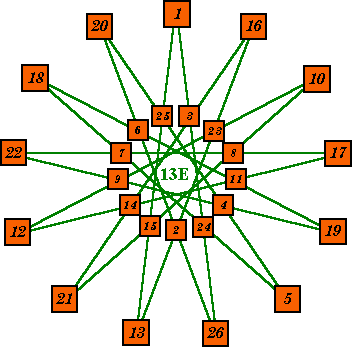This is index #10 of more then 3,000,000 basic solutions for order-13E.### Order-14AOrder-14 uses the numbers from 1 to 28. Each of the 14 lines sums to 58.

There are five different patterns in this order. 3 of  the patterns are non-continuous, the other 2 are continuous.

Pattern 14A is non-continuous. It contains 2 septagons with the points of each summing to the same value.

This is index #10 of more then 7,000,000? basic solutions for order-14A.

### Order-14BPattern 14B is continuous.

This is index #10 of more then 7,000,000? basic solutions for order-14B.### Order-14C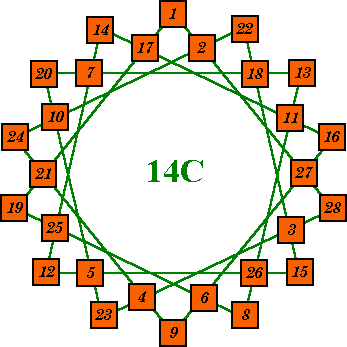Pattern 14C is non-continuous. It consists of 2 type 7A stars with the points of each summing to the same value.

This is index #10 of more then 7,000,000? basic solutions for order-14C.

### Order-14DPattern 14D is a continuous pattern.

This is index #2215000 of more then 7,000,000? basic solutions for order-14D.

Total number of solutions
The numbers given for estimates of the number of basic solutions is just that, an estimate. Because of the large number of solutions, it is impossible to find them all in a reasonable time span. However, time required varies directly with the number of consecutively names points in the pattern. This pattern, with only 2 consecutive point names (AA and BB), runs thousands of times faster then, say, order-14 patterns A, C and E with their 7 consecutive point names.
I started this run on Dec. 24/00 and by Feb. 10/01 had reached solution # 2,215,000. The first number in the solution (cell A) is still 1 and the 2nd number (cell B) now 14. Solution lists for the smaller orders indicate that when the first number becomes a two, approximately one half the solutions have been found.

### Order-14EPattern 14E is non-continuous. It consists of 2 type 7B stars with the points of each summing to the same value.

This is index #10 of more then 7,000,000? basic solutions for order-14E.Harvey Heinz  harveyheinz@shaw.ca
Last updated March 01, 2005
Copyright © 2001 by Harvey D. Heinz